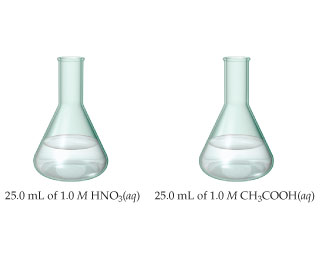# Problem: The samples of nitric and acetic acid shown here are both titrated with a 0.100 M solution of NaOH(aq).Determine whether each of the following statements concerning these titrations is true or false.Phenolphthalein would be a suitable indicator for both titrations.

###### FREE Expert Solution

We are asked to determine if phenolphthalein would be a suitable indicator for the titration of nitric and acetic acid with a 0.100 M solution of NaOH(aq).

An acid-base indicator is a dye that changes color when pH changes.

An indicator is actually a Bronsted-Lowry conjugate acid-base pair in which the acid is a different color to the base.

The endpoint of a titration is when the indicator changes color during titration.

The equivalence point of an acid-base reaction is when the amount of acid and base is just sufficient to cause the complete consumption of both acid and the base.

At the equivalence point, neither acid nor the base is in excess.

At the equivalence point, neither the acid nor the base is the limiting reagent.

The pH of the solution at the equivalence point depends on the relative strength of the acid and the strength of the base used in the titration.

80% (199 ratings)###### Problem Details

The samples of nitric and acetic acid shown here are both titrated with a 0.100 M solution of NaOH(aq).
Determine whether each of the following statements concerning these titrations is true or false.Phenolphthalein would be a suitable indicator for both titrations.